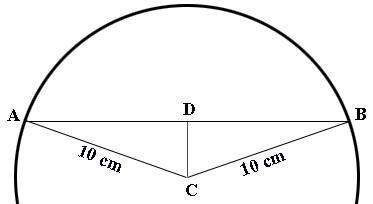SEARCH HOMEMath Central Quandaries & QueriesQuestion from G: A 120 degree central angle intercepts a circle at the points A and B. The radius of the circle is 10 cm. Find the length of chord AB.Hi,

Did you draw a diagram?In my diagram C is the center of the circle and the line CD bisects the angle ACB.

What do you know about triangles DAC and BDC? What does this tell you about the angle BDC?

I hope this helps,
PennyMath Central is supported by the University of Regina and The Pacific Institute for the Mathematical Sciences.